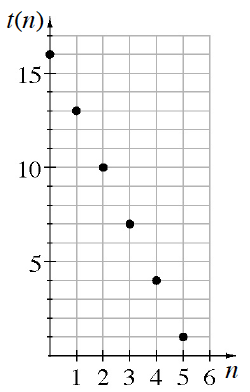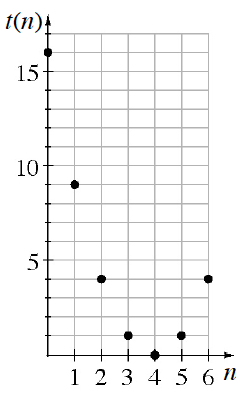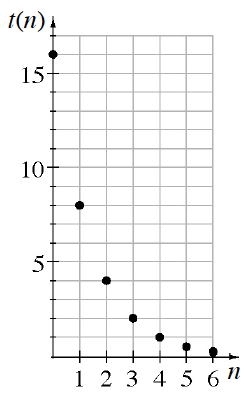### Home > CCAA8 > Chapter 5 Unit 6 > Lesson CCA: 5.3.2 > Problem5-105

5-105.

For this problem, refer to the sequences graphed below.

#$1$#$2$#$3$1. Identify each sequence as arithmetic, geometric, or neither.

Make a list of the $y$-values for each graph. How are these values changing?

Graph #$2$: neither

2. If it is arithmetic or geometric, describe the sequence generator.

• The sequence generator is the number that is being added or multiplied each time.

Your answer for Graph #$3$ should be a factor.